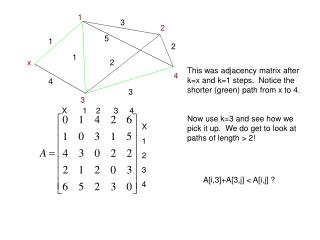DownloadDownload PresentationThis was adjacency matrix after k=x and k=1 steps. Notice the shorter (green) path from x to 4.

# This was adjacency matrix after k=x and k=1 steps. Notice the shorter (green) path from x to 4.

Download Presentation## This was adjacency matrix after k=x and k=1 steps. Notice the shorter (green) path from x to 4.

- - - - - - - - - - - - - - - - - - - - - - - - - - - E N D - - - - - - - - - - - - - - - - - - - - - - - - - - -
##### Presentation Transcript

1. 1 3 2 5 1 2 1 x 2 This was adjacency matrix after k=x and k=1 steps. Notice the shorter (green) path from x to 4. Now use k=3 and see how we pick it up. We do get to look at paths of length > 2! 4 4 3 3 X 1 2 3 4 X 1 2 3 4 A[i,3]+A[3,j] < A[i,j] ?

2. 7.2 Logic circuits • Boolean Expressions • Logic Networks • Truth Functions • Can every truth table be written as a Boolean expression? As a computer circuit?

3. Switches Control (off=false) + - Control (on=true) + -

4. A Or B A + - B

5. A and B A B + -

6. Not A A - +

7. And, Or and Not And ( . ) Or ( + ) Not (‘)

8. Boolean Expressions • A Boolean Expression in n variables x1,x2, .. Xn, is any string of finite symbols formed by the following rules • 1. x1,x2, .. Xn are Boolean expressions • 2. If P and Q are Boolean Expressions, then so are (P+Q), PQ , and P’.

9. Boolean Expressions (x + y) x + y’(xy+z) is a Boolean expression.

10. Truth Functions • A truth function is a truth table for Boolean expressions

11. Find a network for xy’+z X Y Z Exercise: Find a network for (xy’+z)’

12. Find a Boolean expression x y ? z

13. We can do the following Truth function Boolean expression Boolean expression logic network Can we find a Boolean expression for any truth function? Yes!

14. Canonical Form • For the truth function shown, find a Boolean expression: True for: xyz’ xy’z x’yz x’yz’ x’y’z

15. So the truth function is given by: xyz’+xy’z+x’yz+x’yz’+x’y’z This is the Canonical form for the truth function (a standard form into which we can place any two Boolean expressions for comparison.)

16. Simplify, using Boolean algebra: xyz’+xy’z+x’yz+x’yz’+x’y’z = xyz’+xy’z+x’yz+x’yz’+x’y’z+x’yz’ =y’z(x+x’)+x’y(z+z’)+yz’(x+x’) =y’z+x’y+yz’ Notice the groupings of two the same/ one different.